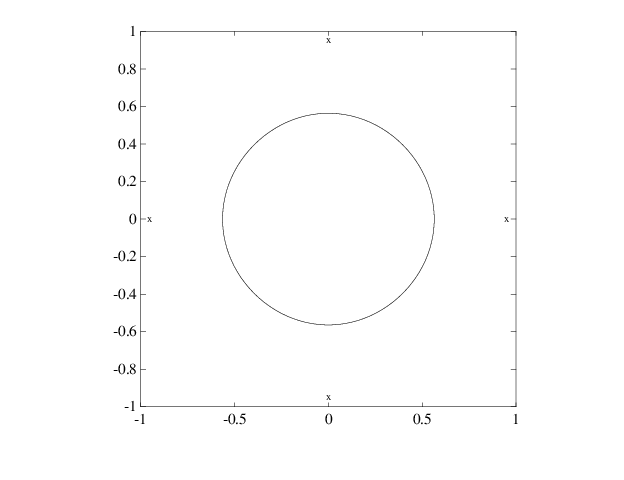## Constructing and Working with B-form Splines

### Construction of B-form

Usually, a spline is constructed from some information, like function values and/or derivative values, or as the approximate solution of some ordinary differential equation. But it is also possible to make up a spline from scratch, by providing its knot sequence and its coefficient sequence to the command `spmak`.

For example, if you enter

```sp = spmak(1:10,3:8); ```

you supply the uniform knot sequence `1:10` and the coefficient sequence `3:8`. Because there are 10 knots and 6 coefficients, the order must be 4(= 10 – 6), i.e., you get a cubic spline. The command

```fnbrk(sp) ```

prints out the constituent parts of the B-form of this cubic spline, as follows:

```knots(1:n+k) 1 2 3 4 5 6 7 8 9 10 coefficients(d,n) 3 4 5 6 7 8 number n of coefficients 6 order k 4 dimension d of target 1 ```

Further, `fnbrk` can be used to supply each of these parts separately.

But the point of the Curve Fitting Toolbox™ spline functionality is that there shouldn't be any need for you to look up these details. You simply use `sp` as an argument to commands that evaluate, differentiate, integrate, convert, or plot the spline whose description is contained in `sp`.

### Working With B-form Splines

The following commands are available for spline work. There is `spmak` and `fnbrk` to make up a spline and take it apart again. Use `fn2fm` to convert from B-form to ppform. You can evaluate, differentiate, integrate, minimize, find zeros of, plot, refine, or selectively extrapolate a spline with the aid of `fnval`, `fnder`, `fndir`, `fnint`, `fnmin`, `fnzeros`, `fnplt`, `fnrfn`, and `fnxtr`.

There are five commands for generating knot sequences:

• `augknt` for providing boundary knots and also controlling the multiplicity of interior knots

• `brk2knt` for supplying a knot sequence with specified multiplicities

• `aptknt` for providing a knot sequence for a spline space of given order that is suitable for interpolation at given data sites

• `optknt` for providing an optimal knot sequence for interpolation at given sites

• `newknt` for a knot sequence perhaps more suitable for the function to be approximated

• `aveknt` to supply certain knot averages (the Greville sites) as recommended sites for interpolation

• `chbpnt` to supply such sites

• `knt2brk` and `knt2mlt` for extracting the breaks and/or their multiplicities from a given knot sequence

To display a spline curve with given two-dimensional coefficient sequence and a uniform knot sequence, use `spcrv`.

You can also write your own spline construction commands, in which case you will need to know the following. The construction of a spline satisfying some interpolation or approximation conditions usually requires a collocation matrix, i.e., the matrix that, in each row, contains the sequence of numbers DrBj,k(τ), i.e., the rth derivative at τ of the jth B-spline, for all j, for some r and some site τ. Such a matrix is provided by `spcol`. An optional argument allows for this matrix to be supplied by `spcol` in a space-saving spline-almost-block-diagonal-form or as a MATLAB® sparse matrix. It can be fed to `slvblk`, a command for solving linear systems with an almost-block-diagonal coefficient matrix. If you are interested in seeing how `spcol` and `slvblk` are used in this toolbox, have a look at the commands `spapi`, `spap2`, and `spaps`.

In addition, there are routines for constructing cubic splines. `csapi` and `csape` provide the cubic spline interpolant at knots to given data, using the not-a-knot and various other end conditions, respectively. A parametric cubic spline curve through given points is provided by `cscvn`. The cubic smoothing spline is constructed in `csaps`.

### Example: B-form Spline Approximation to a Circle

As another simple example,

```points = .95*[0 -1 0 1;1 0 -1 0]; sp = spmak(-4:8,[points points]); ```

provides a planar, quartic, spline curve whose middle part is a pretty good approximation to a circle, as the plot on the next page shows. It is generated by a subsequent

```plot(points(1,:),points(2,:),'x'), hold on fnplt(sp,[0,4]), axis equal square, hold off ```

Insertion of additional control points $\left(±0.95,±0.95\right)/\sqrt{1.9}$ would make a visually perfect circle.

Here are more details. The spline curve generated has the form Σ8j=1Bj,5a(:, j), with -`4:8 `the uniform knot sequence, and with its control points a(:,j) the sequence (0,α),(–α,0),(0,–α),(α,0),(0,α),(–α,0),(0,–α),(α,0) with α=0.95. Only the curve part between the parameter values 0 and 4 is actually plotted.

To get a feeling for how close to circular this part of the curve actually is, compute its unsigned curvature. The curvature κ(t) at the curve point γ(t) = (x(t), y(t)) of a space curve γ can be computed from the formula

`$\kappa =\frac{|x\text{'}y\text{'}\text{'}-y\text{'}x\text{'}\text{'}|}{{\left(x{\text{'}}^{2}+y{\text{'}}^{2}\right)}^{3/2}}$`

in which x', x″, y', and y” are the first and second derivatives of the curve with respect to the parameter used (t). Treat the planar curve as a space curve in the (x,y)-plane, hence obtain the maximum and minimum of its curvature at 21 points as follows:

```t = linspace(0,4,21);zt = zeros(size(t)); dsp = fnder(sp); dspt = fnval(dsp,t); ddspt = fnval(fnder(dsp),t); kappa = abs(dspt(1,:).*ddspt(2,:)-dspt(2,:).*ddspt(1,:))./... (sum(dspt.^2)).^(3/2); [min(kappa),max(kappa)] ans = 1.6747 1.8611 ```

So, while the curvature is not quite constant, it is close to 1/radius of the circle, as you see from the next calculation:

```1/norm(fnval(sp,0)) ans = 1.7864 ```

Spline Approximation to a Circle; Control Points Are Marked `x`